1 Lion data set as 2-sample t-test

Example analysis of the lion data using a two-sample t-test.

1.1 Preliminaries

#The following sets up the data fzo the analysis
#Set working directory

setwd("C:/Users/lisanjie2/Desktop/TEACHING/1_STATS_CalU/1_STAT_CalU_2016_by_NLB/Lecture/Unit3_regression/last_week")

1.2 Plot Lion data

par(mfrow = c(1,1))
plot(age.years ~ portion.black, data = dat,
main = "Lion pigment data split into 3 groups",
ylim = c(0,19))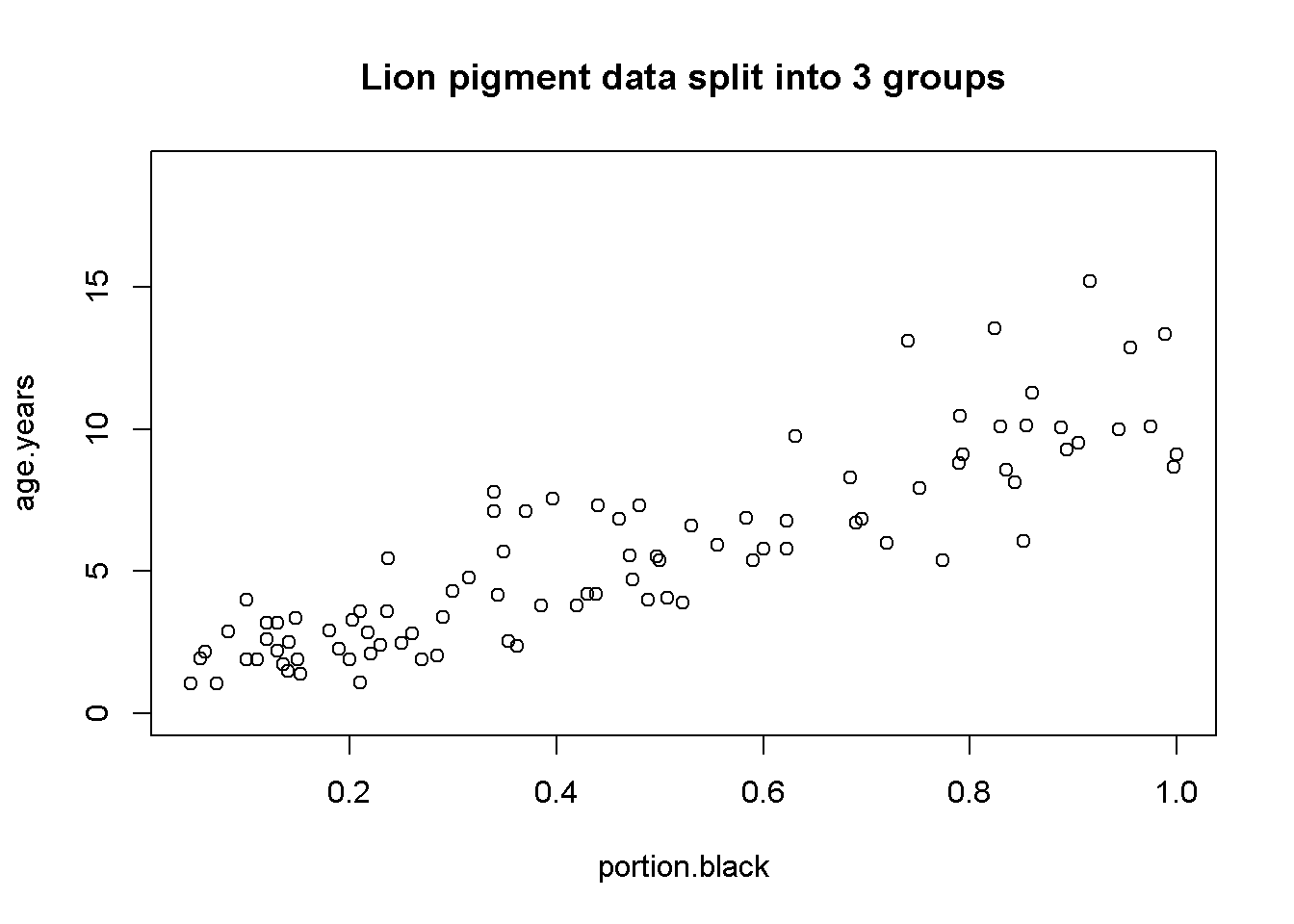1.3 Split lion data by groups

Make two groups, low pigmentation and high pigmentation, by converting the numeric pigmentation variable.

par(mfrow = c(1,1))
pigment.mean <- mean(dat$portion.black) plot(age.years ~ portion.black, data = dat, main = "Lion pigment data split into 2 groups", ylim = c(0,19)) abline(v = pigment.mean, col = 2, lwd = 3) text(x = 0.125, y = 17, "Low\npigment", cex =1.1) text(x = 0.895, y = 17, "High\npigment", cex = 1.1)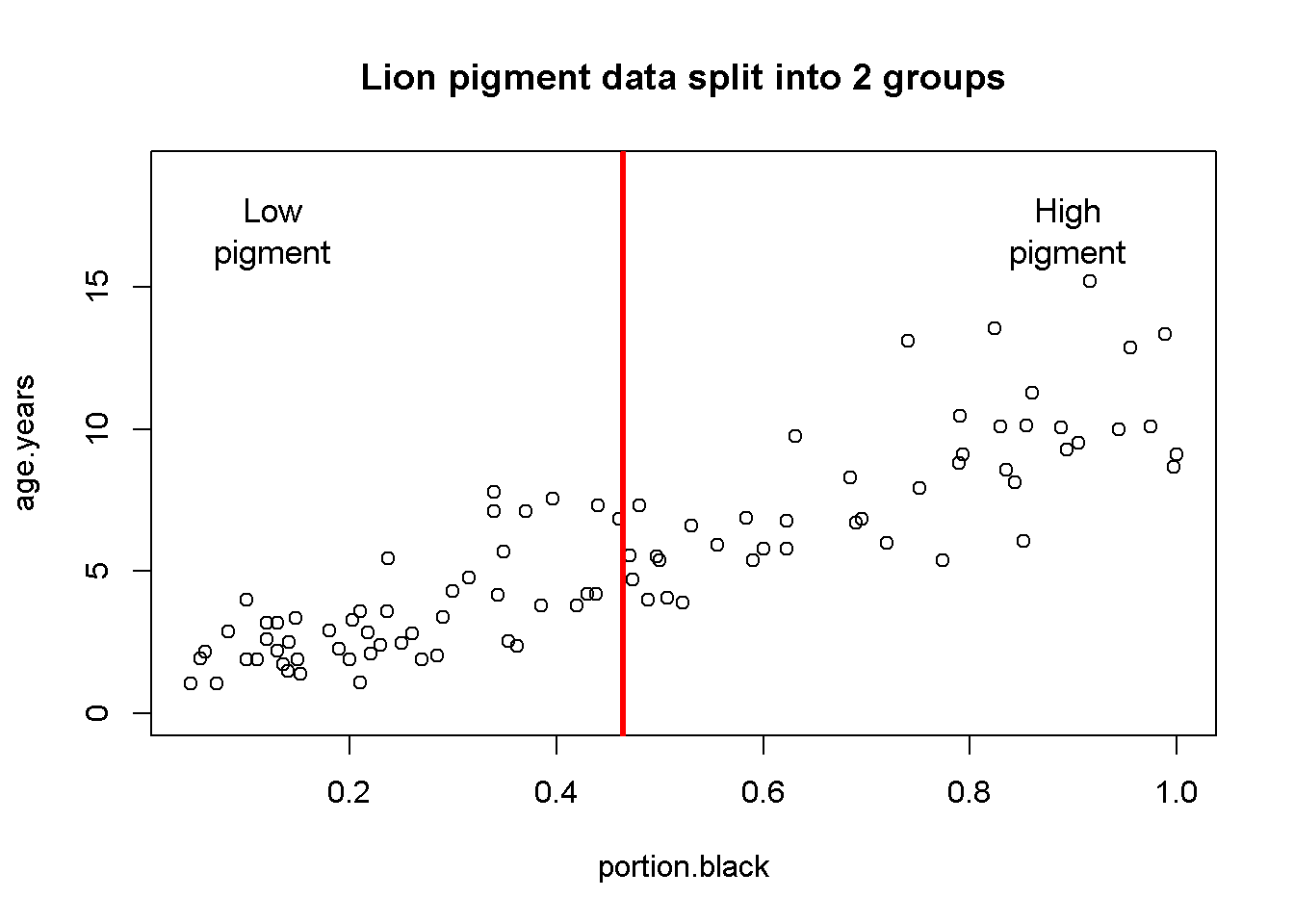1.4 Create Two pigment groups pigment.mean <- mean(dat$portion.black)

dat$pigment.groups.2 <- "high.pigment" dat$pigment.groups.2[which(dat$portion.black <= pigment.mean)] <- "low.pigment" dat$pigment.groups.2 <- factor(dat$pigment.groups.2) summary(dat) ## age.years portion.black population sex ## Min. : 1.056 Min. :0.04609 Ngorogoro:10 female:61 ## 1st Qu.: 2.800 1st Qu.:0.21000 Serengeti:83 male :32 ## Median : 5.378 Median :0.43000 ## Mean : 5.589 Mean :0.46446 ## 3rd Qu.: 7.800 3rd Qu.:0.72000 ## Max. :15.178 Max. :1.00000 ## pigment.groups.2 ## high.pigment:43 ## low.pigment :50 ## ## ## ## dat$pigment.groups.2 <- factor(dat$pigment.groups.2, levels =c("low.pigment", "high.pigment")) table(dat$pigment.groups.2, dat$population) ## ## Ngorogoro Serengeti ## low.pigment 6 44 ## high.pigment 4 39 table(dat$pigment.groups.2, dat\$sex)
##
##                female male
##   low.pigment      25   25
##   high.pigment     36    7

NOTE: Splitting up a continous variable like this into a categorical variable and doing an ANOVA is generally a BAD idea. Regression is the proper way to analyze the raw data. I am making these groups for illustration purposes only!

1.5 Plot raw data by pigmentation group

par(mfrow = c(1,1))
plot(age.years ~ pigment.groups.2,data = dat,
xlab = "Pigmentation group",
ylab = "Known Lion Age")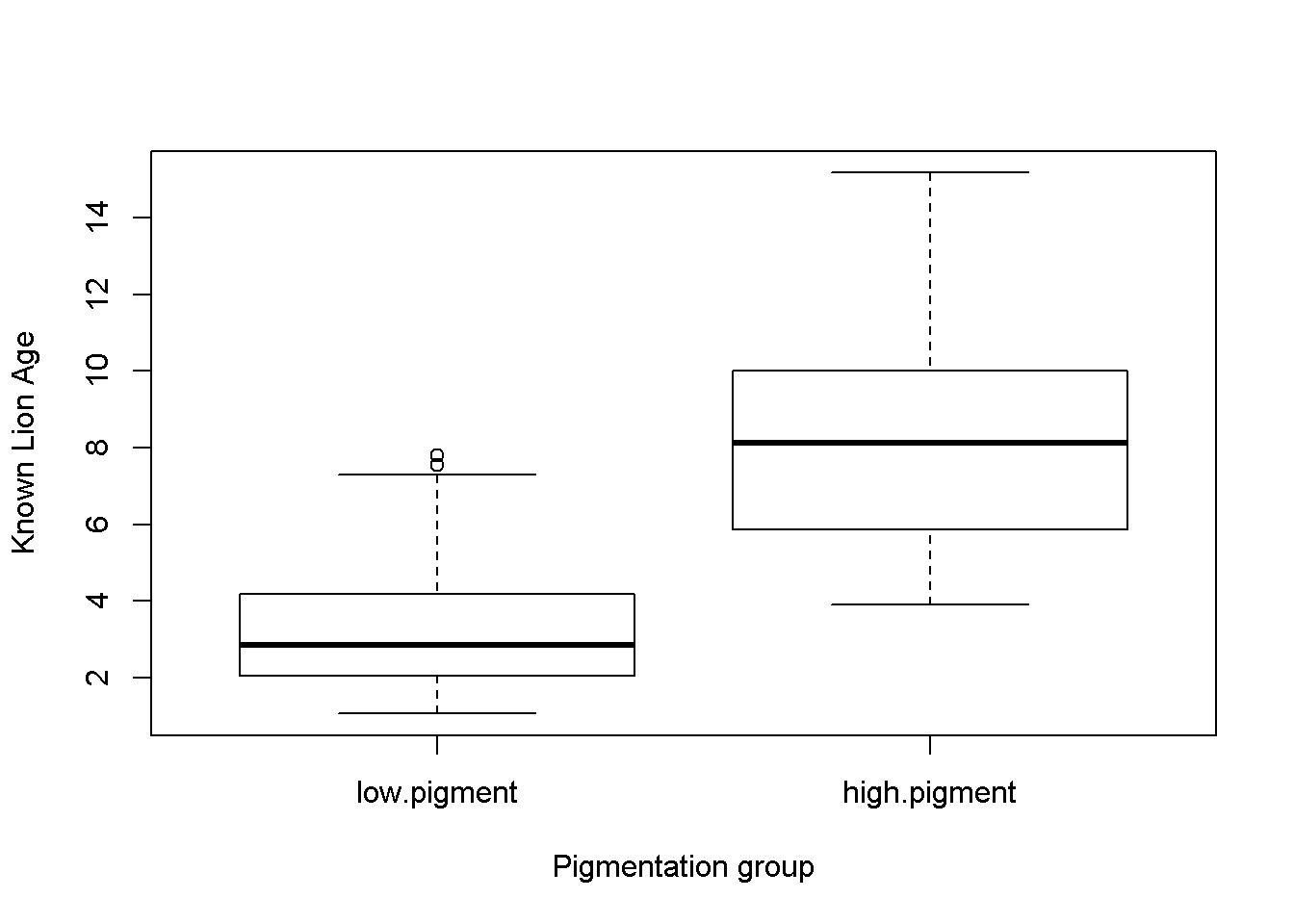Figure 1a: Boxplots of lion ages in two pigmentation groups from the Serengeti and Ngorogoro Crater, Tanzania, east Africa.

1.6 Plot means

library(sciplot)

ci.fun. <- function(x) c(mean(x)-2*se(x), mean(x)+3*se(x))
lineplot.CI(x.factor = pigment.groups.2,
response = age.years,
data = dat,
ci.fun = ci.fun.
)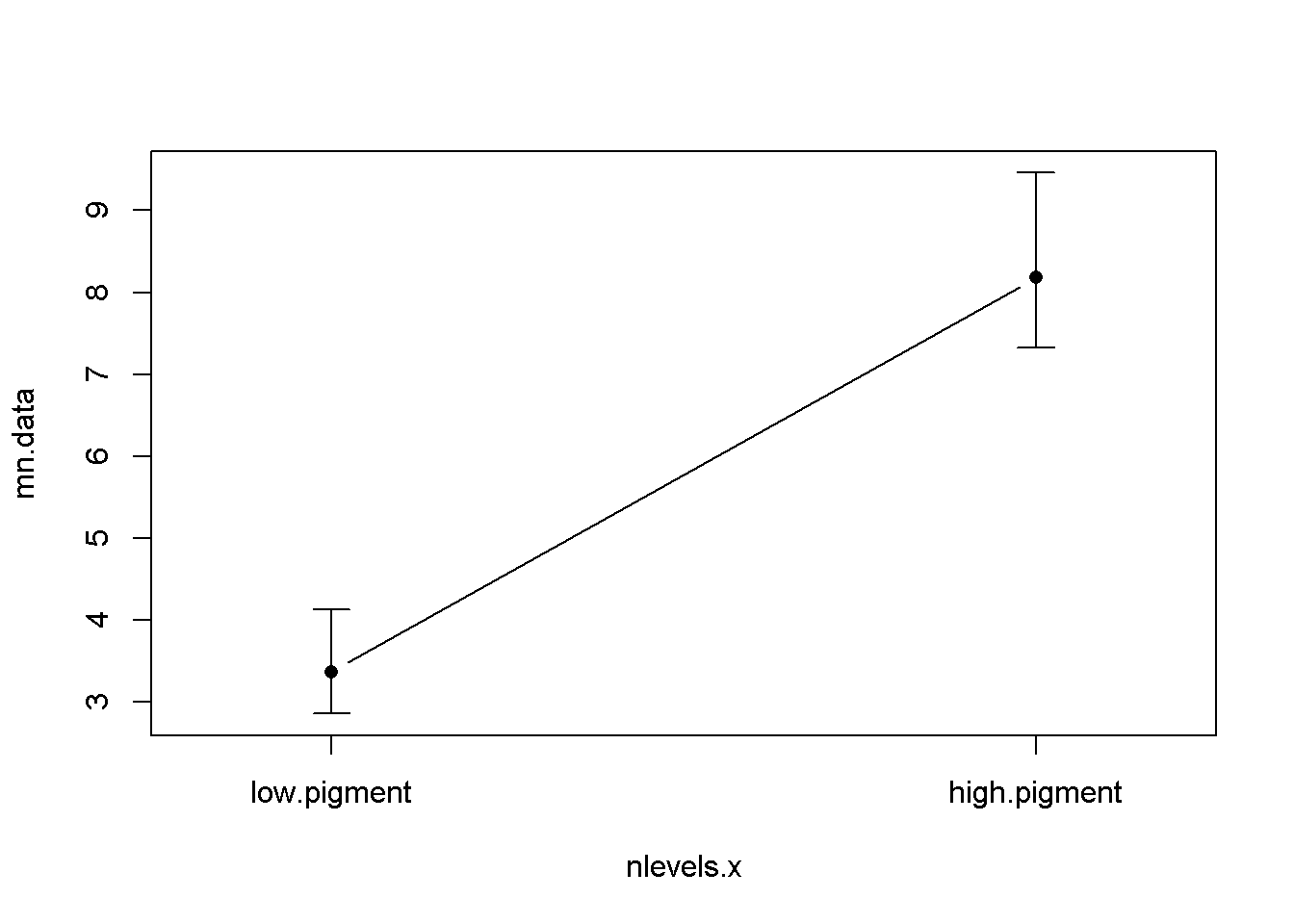Figure 2a: Mean age of lions in two pigmentation groups from the Serengeti and Ngorogoro crater, Tanzania, east Africa. Error bars are approximate 95% confidence intervals.

1.7 2-sample t-test

1.7.1 The Normal way: 2-sample t-test w/ t.test

Test whether the two pigmentation groups have sig. different mean ages.

t.test(age.years ~ pigment.groups.2, data = dat)
##
##  Welch Two Sample t-test
##
## data:  age.years by pigment.groups.2
## t = -9.6798, df = 69.782, p-value = 1.567e-14
## alternative hypothesis: true difference in means is not equal to 0
## 95 percent confidence interval:
##  -5.808789 -3.823945
## sample estimates:
##  mean in group low.pigment mean in group high.pigment
##                   3.362444                   8.178811

1.7.2 The alternative way: t-test with lm()

You can set up basically the same test/model using the function lm() (though note that Welch’s correction for unequal variances won’t be applied). Doing this is a useful way to look at the residuals of the t-test.

1.7.2.1 Fit lm() model

lm.t.test <- lm(age.years ~ pigment.groups.2, data = dat)

1.7.2.2 Look at diagnostics of the t-test

Get the residuals

resids <- resid(lm.t.test)

Plot diagnostic plots

par(mfrow = c(2,2))
hist(resids)
plot(lm.t.test, which = 2)
plot(lm.t.test, which = 1)
plot(lm.t.test, which = 5)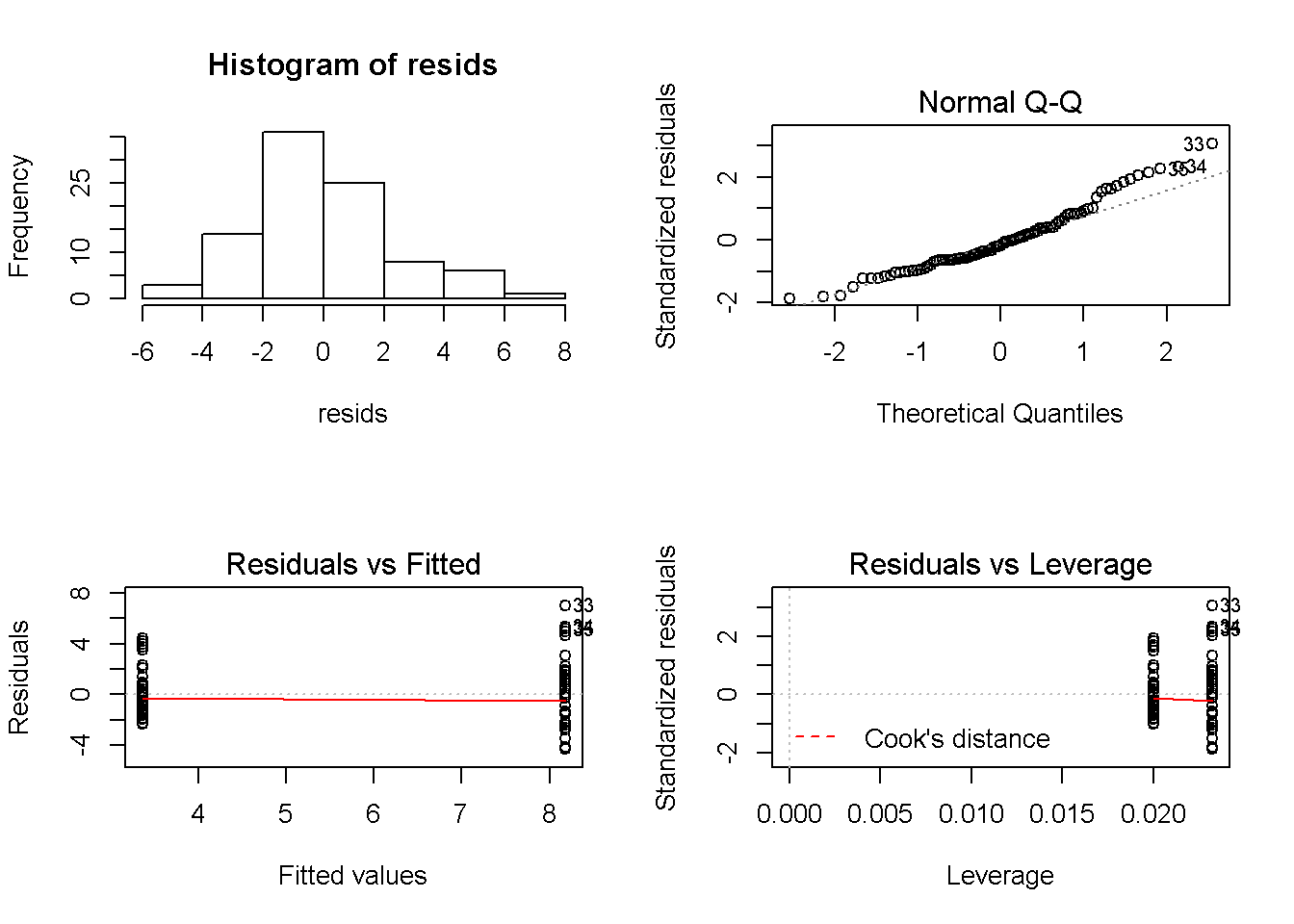• Normal QQ plot is a little off
• Residual’s vs. fitted indicates some difference in variance between the groups

1.7.2.3 Fit lm() model to logged data

Check if log transformation improves thigns

lm.t.test.log <- lm(log(age.years) ~ pigment.groups.2, data = dat)

1.7.2.4 Look at diagnostics of the t-test

Get the residuals

resids.log <- resid(lm.t.test.log)

Plot diagnostic plots

par(mfrow = c(2,2))
hist(resids.log)
plot(lm.t.test.log, which = 2)
plot(lm.t.test.log, which = 1)
plot(lm.t.test.log, which = 5)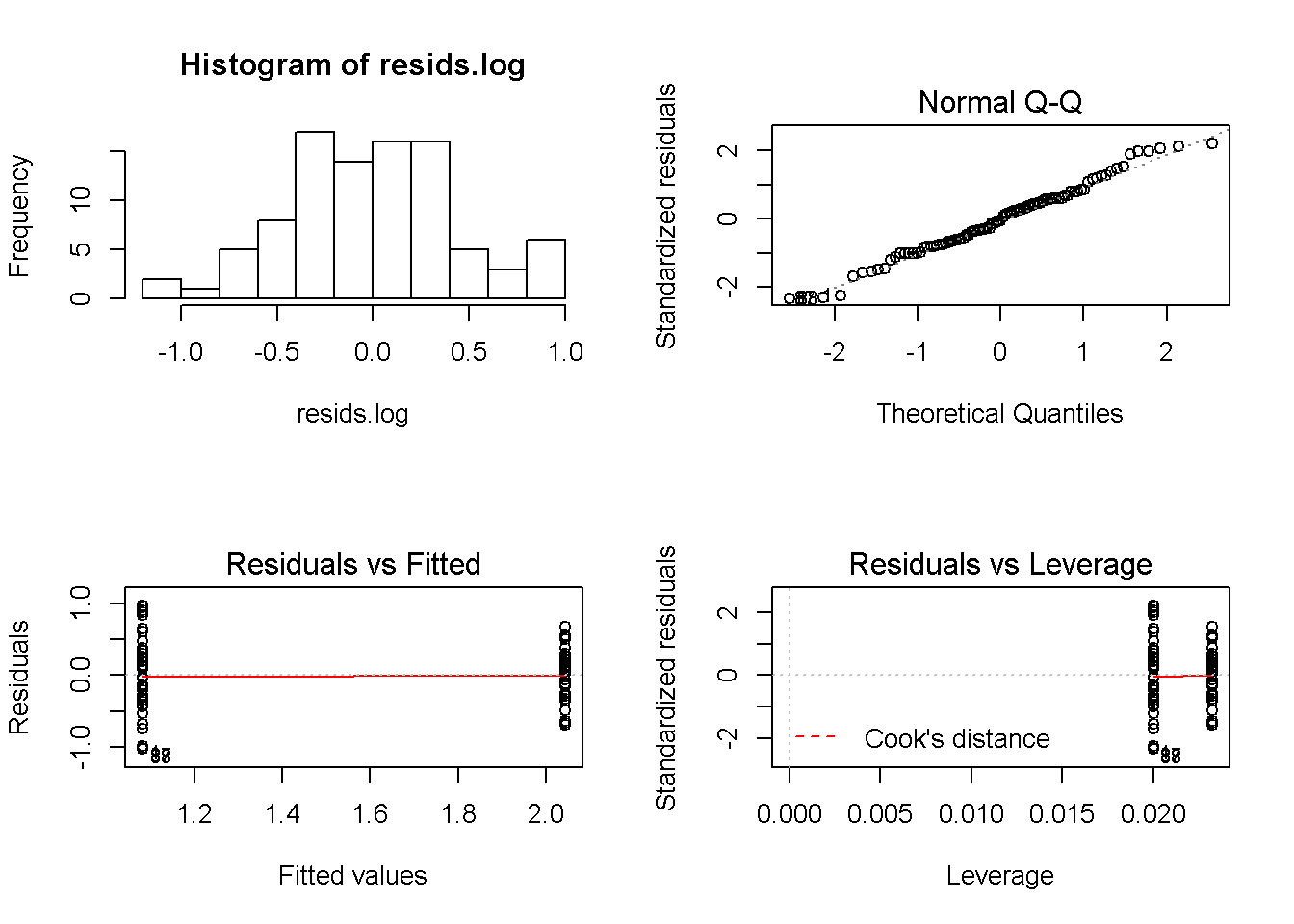• QQ is better
• Residvuals vs. fit is perhaps a little better.